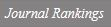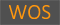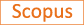Bayesian model selection and parameter estimation for possibly asymmetric and non-stationary time series using a reverisble jump Markov chain Monte Carlo approach

Title
Bayesian model selection and parameter estimation for possibly asymmetric and non-stationary time series using a reverisble jump Markov chain Monte Carlo approach
Authors
Oh M.-S.Shin D.W.
Ewha Authors
SCOPUS Author ID
오만숙; 신동완Issue Date
2002
Journal Title
Journal of Applied Statistics
ISSN
0266-4763Citation
Journal of Applied Statistics vol. 29, no. 5, pp. 771 - 789
Indexed
SCIE; SCOPUSDocument Type
Article
Abstract
A Markov chain Monte Carlo (MCMC) approach, called a reversible jump MCMC, is employed in model selection and parameter estimation for possibly non-stationary and non-linear time series data. The non-linear structure is modelled by the asymmetric momentum threshold autoregressive process (MTAR) of Enders &amp; Granger (1998) or by the asymmetric self-exciting threshold autoregressive process (SETAR) of Tong (1990). The non-stationary and non-linear feature is represented by the MTAR (or SETAR) model in which one (p1) of the AR coefficients is greater than one, and the other (p2) is smaller than one. The other non-stationary and linear, stationary and non-linear, and stationary and linear features, represented respectively by (p1 = p2 = 1), (p1 ≠ p2 &lt; 1) and (p1 = p2 &lt; 1), are also considered as possible models. The reversible jump MCMC provides estimates of posterior probabilities for these four different models as well as estimates of the AR coefficients p1 and p2. The proposed method is illustrated by analysing six series of US interest rates in terms of model selection, parameter estimation, and forecasting.
DOI
10.1080/02664760120098829
Appears in Collections:
자연과학대학 > 통계학전공 > Journal papers
Files in This Item:
There are no files associated with this item.
Export
RIS (EndNote)
XLS (Excel)
XML